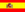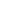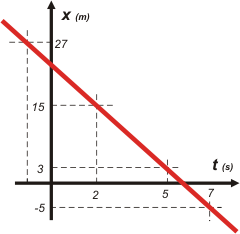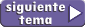home | más de cinemática | otros temas de Física | lecciones del maestro Ciruela | tonterías | @NO ME SALEN
PROBLEMAS RESUELTOS DE FÍSICA DEL CBCUSING A KINETIC EQUATION

Let´s begin with the same equation I used as an example to explain what is a kinetic equation

x = 3 m – 4 m/s ( t  – 5 s )

Just like assembling the kinetic equation means to replace the constants, using a kinetic equation means to replace the variables: X and t. This way we can find out where the movil is in the instant you choose. For example: where is the movil in the instant 74.25 s? Even if you think this question is ridiculous, you can find the answer using the equation. The equation always answer in a docile manner, it will never get offended. To make the equation answer, the variable t is replaced by the instant you want to know about (74.25 s), then, you solve the little account you have, the result of that account is where the movil is in that instant.

You can ask the equation about any instant you want, and the equation will answer precisely. The questions and answers can be put down on a list (value table)

usar o (como dicen los físicos) especializar una ecuación horaria significa reemplazar las variables:
x y t.

 t (s) x (m) 5 3 7 -5 2 15 74,25 -274,04 -1 27

The instants 5; 7; 2; 74.25 and -1 seconds are there because I wanted to put them, but you can put the instants you want too, the equation will answer. And that´s how we can keep asking for data on any instant we want to know about. All the answers are insidethe equation.

By the way... how many space-time pairs that belong to he movement described by this equation do you think are stored in the equation? Exactly! (you were thinking infinite, right?).
Also, hae you noticed that I asked about the instant (-1s)? Was I wrong? Does such a thing even exist? Of course it does, there is nothing wrong about them, they don´t  spread any diseases, nor they have less civil rights than the positive time periods. If you have any doubt about that, you can ask in the negative time.

Now that we some space-time pairs, we might as well make a graph of the movement.Note: when you are using a kinetic equation, don´t even think about changing, or touching the constants, If you do, you are changing the equation, and now it will describe a different movement (you are screwed).

I´ll tell it again, in technicolor this time:

x = 3 m – 4 m/s ( t  – 5 s )

TO USE a kinetic equation, means to REPLACE THE VARIABLES (the red ones; don´t touch the blue ones, they are poop).Some rights reserved. Reproduction allowe by quoting the source. Translated by Santi Pisci. Last Updated jul-15. Buenos Aires, Argentina.